### SYNTHESIS

Based on chapter 10 in The Way of Z.

Links to more Z lectures.

This page looks best when this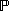and this X are about the same size:X. See these viewing tips.

Sets (functions, relations, ...) and logic are all we need to write small formal descriptions.

Well-chosen notational conventions can make mathematical texts easier to read.

Formal descriptions are not just paraphrased natural language. Writing useful formal descriptions requires design judgment.

SET COMPREHENSION

Combine declaration, predicate, expression.

 {  declaration | predicateexpression  }

can be thought of this way

 {  source | filterpattern  }

The expression and predicate are optional.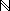== {  i: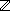| i0  }

 ODD == { i:2*i+1 }

If the expression is the characteristic tuple, it can be omitted.

 line == {  x,y:| y = m*x+b  }

means the same as

 line == {  x,y:| y = m*x+b(x,y)  }

SPECIFICATIONS

Set comprehensions are specifications in miniature.

A prime number is an integer larger than 1 that is only divisible by itself and 1. Informally,

 PRIME == { 2, 3, 5, 7, 11, 13, 17, ... }

Paraphrasing the English

 PRIME == {  n:| n > 1(m: 2 .. n-1n mod m = 0)  }

Can't we do better? A prime is not a product of numbers larger than 1.2 ==\ { 0, 1 }PRIME ==2 \ {  n,m:2n * m  }

We can make specifications clearer by moving away from a literal paraphrase of the English, and using set operators instead of negation and quantification.

LAMBDA EXPRESSION

Lambda expressions define functions.

 (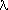declaration | predicateexpression)

 isqr == (i:i*i)

means the same as this set comprehension.

 isqr == {  i:i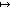i*i  }

Both mean the same asisqr: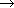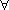i:isqr i = i*iLambda expressions enable us to use functions without writing declarations.

 9 = (i:i*i) 3

RESTRICTED QUANTIFIERS

Restricted quantifiers abbreviate common predicates.i: ns | odd(i)inmax

meansi: nsodd(i)inmax

andi: ns | odd(i)inmax

meansi: nsodd(i)inmax

In general(d | pq)(dpq)(d | pq)(dpq)

CONVENIENCES AND SHORTCUTS

Local definitions factor out common expressions.

 (let r == iroot(a)r*ra < (r+1)*(r+1))

means

 iroot(a) * iroot(a)a < (iroot(a)+1) * (iroot(a)+1)

Conditional expressions simplify two-way case analyses.

 | x | = if x0 then x else -x

means

 (x0| x | = x)(x < 0| x | = -x)

Back to Z lectures.

Jonathan Jacky / University of Washington / Seattle, Washington / USA
E-mail: jon@u.washington.edu